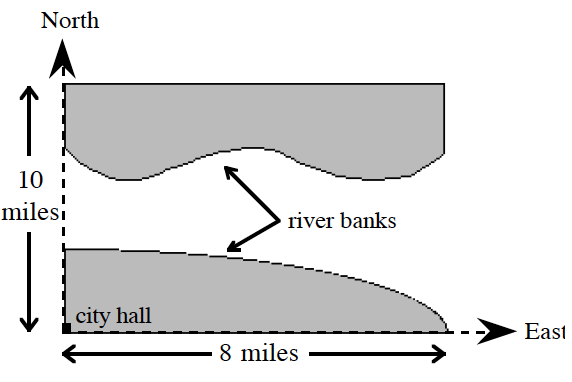### Home > CALC > Chapter 7 > Lesson 7.2.3 > Problem7-89

7-89.

Remember our city that straddles the Newton River? It is applying for National Parks and Recreation funds and needs to know the area of land (shaded in the diagram) within the city limits.

Given the equations of the river banks, determine the area of land.

North Bank: $y =\operatorname{cos}( \frac { \pi x } { 2 } )+ 6$
South Bank: $y =\operatorname{ln}(9 − x)$The $x$-axis is the line pointing east, and the $y$-axis is the line pointing north.

Set up an integral to find the area of the Northern region first.
Note that this will be the area between a horizontal line (on top) and a curvy line (on the bottom).

The area of the Southern region will be easier to set up.